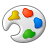# Summary Statistic Tool - Weighted average

457
1
05-27-2014 11:21 PM
Status: Open
Labels (1)Occasional Contributor

Give us the possibility in the summary statistics tool to calculate weighted averages, where the weights are taken from another field. Currently the tool only allows to calculate simple averages (among other statistics like min, max, mean etc.). The corresponding statistics tool in ArcInfo Workstation allows calculation of weighted averages since the beginning, so I am wondering why the summary statistics tool is lacking such option.

Tags (1)
1 CommentWell, here's a good idea that has seen no activity. Yes, a weighted average calculator would be great...and specifically an area-weighted average would be a nice touch. so how about...

- weighted average

- area-weighted average

- most frequent occurrence

Please make summary statistics as expansive and useful as possible.